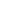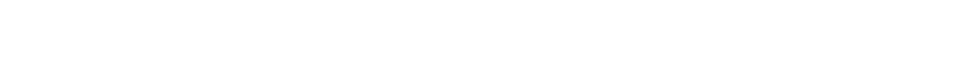User Rating: 5 / 5So I have decided to make public the most useful SQL queries I have developed while dealing with FusionPBX. Please note that I am a MariaDB user, I have no intention to translate to PostgreSQL for free. Remember to change them to fit your specific needs.

## Call Lenght Statistic

Since many crapy carriers have been starting to charge for short calls, it is important to monitor and know this. This query will give you an insight into this:

SELECT accountcode, AVG(billsec) AS average, SUM(CASE WHEN billsec = 0 THEN 1 ELSE 0 END) AS zero_calls, SUM(CASE WHEN billsec BETWEEN 1 AND 6 THEN 1 ELSE 0 END) AS short_calls, SUM(CASE WHEN billsec BETWEEN 1 AND 60 THEN 1 ELSE 0 END) AS medium_calls, SUM(CASE WHEN billsec > 6 THEN 1 ELSE 0 END) AS long_calls, SUM(CASE WHEN billsec > 0 THEN 1 ELSE 0 END) AS total_answered_calls, SUM(1) AS total_calls FROM v_xml_cdr  WHERE  start_stamp >= DATE_SUB(NOW(), INTERVAL 30 DAY) GROUP BY accountcode;Where:

• average is the total billsec average. Null values are ignored,
• zero_calls is the total or unanswered calls for any reason,
• short_calls is the total of answered calls with a duration between 1 and 6 seconds,
• medium_calls is the total of answered calls with a duration between 1 and 60 seconds. This value includes short_calls.
• long_calls is the total of any answered call with a duration longer than 6 seconds. This value includes some values from the medium_calls metric.
• total_answered_calls is the total of any answered call regardless of the duration, and
• total_calls is the total of calls regardless if the call was answered or not.

## Calls by Carrier

If you are using more than one carrier you can label the call to have some statistics. I do mark the calls with my LCR for FusionPBX. The following query helps to know some useful information:

SELECT JSON_VALUE(json,'\$.variables.lcr_carrier') AS json_carrier, COUNT(*) AS c, SUM(call_sell) AS sold, SUM(call_buy) AS bought FROM v_xml_cdr WHERE  start_stamp >= DATE_SUB(NOW(), INTERVAL 30 DAY) GROUP BY json_carrier;Where:

• json_carrier is the carrier name I am routing through,
• c is the total of calls routed,
• sold is the total revenue by the minute, and
• bought is the total purchase

This query assumes all the records and carriers are using the same query.

## Simultaneous Calls Statistics

As the VoIP business grows, servers will need more capacity. Knowing how many simultaneous calls you can handle is a very important matter. The following query will give statistics about the simultaneous calls you can handle.

SELECT YEAR(start_stamp) y, MONTH(start_stamp) m, MIN(c), AVG(c), MAX(c), STDDEV(c) FROM (SELECT start_epoch, start_stamp, COUNT(*) AS c FROM v_xml_cdr WHERE  start_stamp >= DATE_SUB(NOW(), INTERVAL 30 DAY) AND ((start_epoch BETWEEN start_epoch AND end_epoch) OR (end_epoch BETWEEN start_epoch AND end_epoch)) GROUP BY start_epoch ) a GROUP BY y, m ORDER BY y DESC, m DESC;Where:

• y is the year,
• m is the month,
• MIN(c) is the minimum number of simultaneous calls that have been in your PBX when there was at least one call,
• AVG(c) is the arithmetic mean of the number of calls in your PBX,
• MAX(c) the maximum number of simultaneous calls you have had, and
• STDDEV(c) the standard deviation.

## VoIP Metrics: ASR & ALOC

ASR and ALOC are two metrics that are used to know if a carrier is failing or if a customer is misbehaving. The following query will give you these metrics:

SELECT accountcode, SUM(CASE WHEN billsec > 0 THEN 1 ELSE 0 END) / sum(1) * 100 AS asr, SUM(billsec) / SUM(CASE WHEN billsec > 0 THEN 1 ELSE 0 end) AS aloc FROM v_xml_cdr WHERE start_stamp >= DATE_SUB(NOW(), INTERVAL 30 DAY) GROUP BY accountcode;Where:

• asr is the ASR, and
• aloc is the ALOC.

## Price Analysis

If you are using my LCR for FusionPBX, this query gives an insight into whom is your better carrier with some price statistics.

SELECT destination, description, MIN(lcr_rate), AVG(lcr_rate), MAX(lcr_rate), STDDEV(lcr_rate), GROUP_CONCAT(lcr_carrier_name ORDER BY lcr_rate) FROM (SELECT  1809327 as destination, l.description, c.carrier_name as lcr_carrier_name, l.rate as lcr_rate, l.connect_rate as lcr_second_rate  FROM v_lcr l JOIN v_carriers c ON l.carrier_uuid=c.carrier_uuid  WHERE c.enabled = 'true' AND l.enabled = 'true' AND l.lcr_direction = 'outbound' AND NOW() >= l.date_start AND NOW() < l.date_end AND l.digits = (SELECT MAX(digits) AS max FROM v_lcr lll WHERE lll.enabled='true' AND lll.digits IN ('1809327', '180932', '18093', '1809', '180', '18', '1') AND lll.lcr_direction = 'outbound' AND lll.carrier_uuid = c.carrier_uuid)  ORDER BY c.priority ASC, lcr_rate ASC, l.digits DESC,  l.date_start DESC) a;Where:

• destination is the number you want to know about,
• description is your note,
• MIN(lcr_rate) is the minimum buying price,
• AVG(lcr_rate) is the average buying price,
• MAX(lcr_rate) is the maximum buying price, and
• GROUP_CONCAT(...) is the list of carriers who serve that destination ordered by rate.

There is not an easy way to query all the destinations at once, especially because of the lack of a function to create the series for the IN clause.

I will add more queries as I create them.

Good luck!